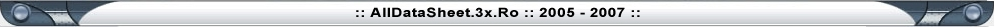Home

Resistors

Resistors are the most commonly used component in electronics and their purpose is to create specified values of  current and voltage in a circuit. A number of different resistors are shown in the photos. (The resistors are on millimeter paper, with 1cm spacing to give some idea of the dimensions).  Photo 1.1a shows some low-power resistors, while photo 1.1b shows some higher-power resistors. Resistors with power dissipation below 5 watt (most commonly used types) are cylindrical in shape, with a wire protruding from each end for connecting to a circuit (photo 1.1-a). Resistors with power dissipation above 5 watt are shown below (photo 1.1-b).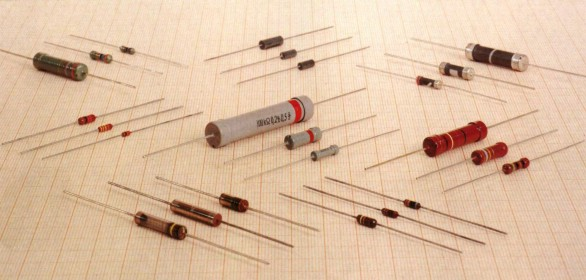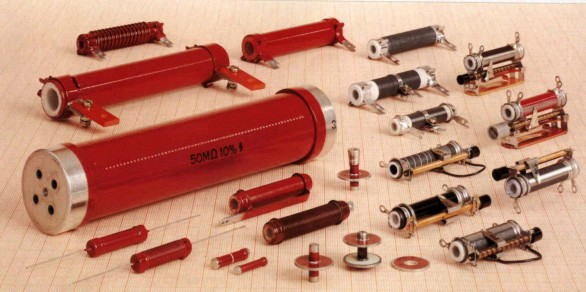Fig. 1.1a: Some low-power resistors Fig. 1.1b: High-power resistors and rheostats

The symbol for a resistor is shown in the following diagram (upper: American symbol, lower: European symbol.)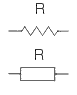Fig. 1.2a: Resistor symbols

The unit for measuring resistance is the OHM. (the Greek letter Ω). Higher resistance values are represented by "k" (kilo-ohms) and M (meg ohms). For example, 120 000 Ω is represented as 120k, while 1 200 000 Ω is represented as 1M2. The dot is generally omitted as it can easily be lost in the printing process. In some circuit diagrams, a value such as 8 or 120 represents a resistance in ohms. Another common practice is to use the letter E for resistance. For example, 120E (120R) stands for 120 Ω, 1E2 stands for 1R2 etc.

1. Resistor Markings

Resistance value is marked on the resistor body. The first three bands provide the value of the resistor in ohms and the fourth band indicates the tolerance. Tolerance values of  5%, 2%, and 1% are most commonly available.

The following table shows the colors used to identify resistor values:

 COLOR DIGIT MULTIPLIER TOLERANCE TC Silver x 0.01 W ±10% Gold x 0.1 W ±5% Black 0 x 1 W Brown 1 x 10 W ±1% ±100*10-6/K Red 2 x 100 W ±2% ±50*10-6/K Orange 3 x 1 kW ±15*10-6/K Yellow 4 x 10 kW ±25*10-6/K Green 5 x 100 kW ±0.5% Blue 6 x 1 MW ±0.25% ±10*10-6/K Violet 7 x 10 MW ±0.1% ±5*10-6/K Grey 8 x 100 MW White 9 x 1 GW ±1*10-6/K

** TC - Temp. Coefficient, only for SMD devices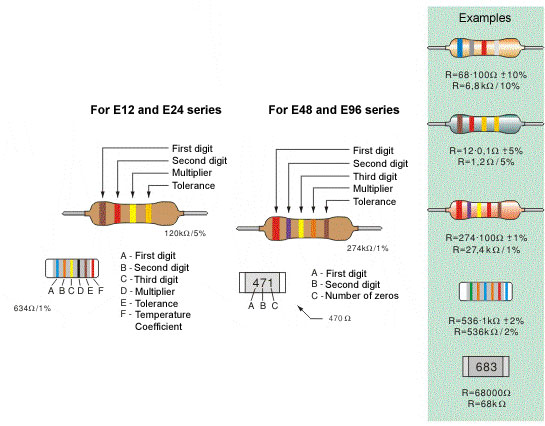Fig. 1.2: b. Four-band resistor, c. Five-band resistor, d. Cylindrical SMD resistor, e. Flat SMD resistor

The following shows all resistors from 1R to 22M: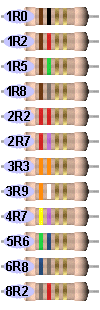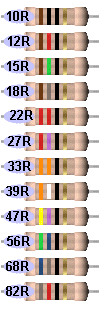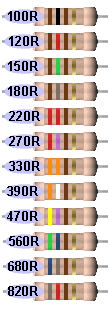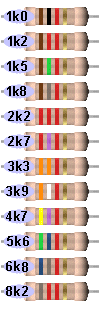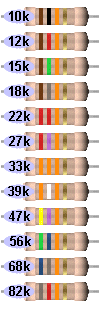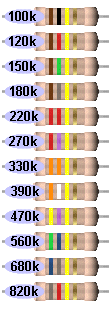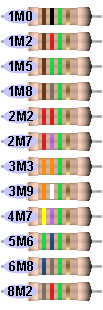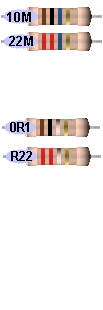NOTES:
The resistors above are "common value"  5% types.
The fourth band is called the "tolerance" band. Gold = 5%
(tolerance band Silver =10% but no modern resistors are 10%!!)
"common resistors" have values 10 ohms to 22M.

RESISTORS LESS THAN 10 OHMS
When the third band is gold, it indicates the value of the "colors" must be divided by 10.
Gold = "divide by 10" to get values 1R0 to 8R2
See 1st Column above for examples.

When the third band is silver, it indicates the value of the "colors" must be divided by 100.
(Remember: more letters in the word "silver" thus the divisor is "larger.")
Silver = "divide by 100" to get values R1 to R82
e.g: 0R1 = 0.1 ohm     0R22 =  point 22 ohms
See 4th Column above for examples.

The letters "R, k and M" take the place of a decimal point.
e.g: 1R0 = 1 ohm     2R2 = 2 point 2 ohms   22R = 22 ohms
2k2 = 2,200 ohms     100k = 100,000 ohms
2M2 = 2,200,000 ohms

Common resistors have 4 bands. These are shown above. First two bands indicate the first two digits of the resistance, third band is the multiplier (number of zeros that are to be added to the number derived from first two bands) and fourth represents the tolerance.

Marking the resistance with five bands is used for resistors with tolerance of 2%, 1% and other high-accuracy resistors. First three bands determine the first three digits, fourth is the multiplier and fifth represents the tolerance.

For SMD (Surface Mounted Device) the available space on the resistor is very small. 5% resistors use a 3 digit code, while 1% resistors use a 4 digit code.

Some SMD resistors are made in the shape of small cylinder while the most common type is flat. Cylindrical SMD resistors are marked with six bands - the first five are "read" as with common five-band resistors, while the sixth band determines the Temperature Coefficient (TC), which gives us a value of resistance change upon 1-degree temperature change.

The resistance of flat SMD resistors is marked with digits printed on their upper side. First two digits are the resistance value, while the third digit represents the number of zeros. For example, the printed number 683 stands for 68000W , that is 68k.

It is self-obvious that there is mass production of all types of resistors. Most commonly used are the resistors of the E12 series, and have a tolerance value of 5%. Common values for the first two digits are: 10, 12, 15, 18, 22, 27, 33, 39, 47, 56, 68 and 82.
The E24 series includes all the values above, as well as: 11, 13, 16, 20, 24, 30, 36, 43, 51, 62, 75 and 91. What do these numbers mean?  It means that resistors with values for digits "39" are: 0.39W, 3.9W, 39W, 390W, 3.9kW, 39kW, etc are manufactured. (0R39, 3R9, 39R, 390R, 3k9, 39k)

For some electrical circuits, the resistor tolerance is not important and it is not specified. In that case, resistors with 5% tolerance can be used. However, devices which require resistors to have a certain amount of accuracy, need a specified tolerance.

2. Resistor Dissipation

If the flow of current through a resistor increases,  it heats up, and if the temperature exceeds a certain critical value, it can be damaged. The wattage rating of a resistor is the power it can dissipate over a long period of time.
Wattage rating is not identified on small resistors. The following diagrams show the size and wattage rating: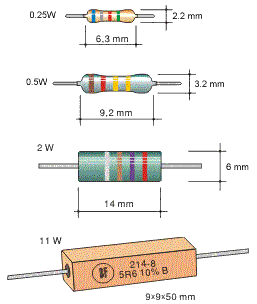Fig. 1.3: Resistor dimensions

Most commonly used resistors in electronic circuits have a wattage rating of 1/2W or 1/4W. There are smaller resistors  (1/8W and 1/16W) and higher (1W, 2W, 5W, etc).
In place of a single resistor with specified dissipation, another one with the same resistance and higher rating may be used, but its larger dimensions increase the space taken on a printed circuit board as well as the added cost.

Power (in watts) can be calculated according to one of the following formulae: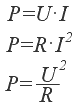where V represents resistor voltage in Volts, I is the current flowing through the resistor in Amps and R is the resistance of resistor in Ohms. For example, if the voltage across an 820W resistor is 12V, the wattage dissipated by the resistors is: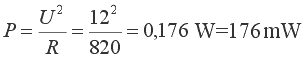A 1/4W resistor can be used.

In many cases, it is not easy to determine the current or voltage across a resistor. In this case the wattage dissipated by the resistor is determined for the "worst" case. We should assume the highest possible voltage across a resistor, i.e. the full voltage of the power supply (battery, etc).
If we mark this voltage as VB, the lowest dissipation is: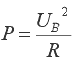For example, if VB=9V, the dissipation of a 220W resistor is: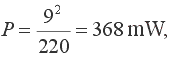A 0.5W or higher wattage resistor should be used

3. Nonlinear resistors

Resistance values detailed above are a constant and do not change if the voltage or current-flow alters. But there are circuits that require resistors to change value with a change in temperate or light. This function may not be linear, hence the name NONLINEAR RESISTORS.

There are several types of nonlinear resistors, but the most commonly used include : NTC resistors (figure a) (Negative Temperature Co-efficient) - their resistance lowers with temperature rise. PTC resistors (figure b) (Positive Temperature Co-efficient) - their resistance increases with the temperature rise. LDR resistors (figure c) (Light Dependent Resistors) - their resistance lowers with the increase in light. VDR resistors (Voltage dependent Resistors) - their resistance critically lowers as the voltage exceeds a certain value. Symbols representing these resistors are shown below.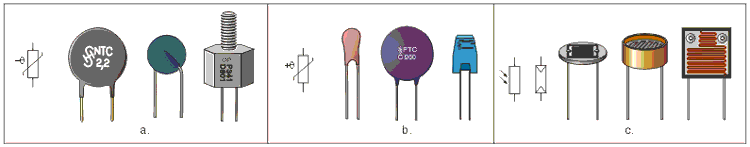Fig. 1.4: Nonlinear resistors - a. NTC, b. PTC, c. LDR

 In amateur conditions where nonlinear resistor may not be available, it can be replaced with other components. For example, NTC resistor may be replaced with a transistor with a trimmer potentiometer, for adjusting the required resistance value. Automobile light may play the role of PTC resistor, while LDR resistor could be replaced with an open transistor. As an example, figure on the right shows the 2N3055, with its upper part removed, so that light may fall upon crystal plate.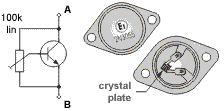4. Practical examples with resistors

Figure 1.5 shows two practical examples with nonlinear and regular resistors as trimmer potentiometers, elements which will be covered in the following chapter.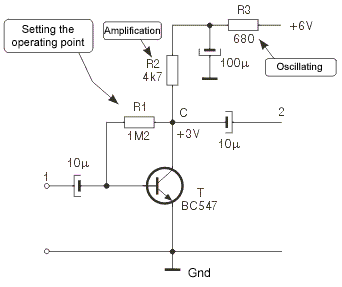Fig. 1.5a: RC amplifier

Figure 1.5a represents the so called RC voltage amplifier, that can be used for amplifying low-frequency, low-amplitude audio signals, such as microphone signal. Signal to be amplified is brought between node 1 and gnd (amplifier input), while the resulting amplified signal appears between node 2 and gnd (amplifier output). To get the optimal performance (high amplification, low distortion, low noise, etc) , it is necessary to "set" the transistor's operating point. Details on operating point will be provided in chapter 4; for now, let's just say that DC voltage between node C and gnd should be approximately one half of battery (power supply) voltage. Since battery voltage equals 6V, voltage in node C should be set to 3V. Adjustments are made via resistor R1.

Connect the voltmeter between node C and gnd. If voltage exceeds 3V, replace the resistor R1=1.2MW with another, smaller resistor, say R1=1MW. If voltage still exceeds 3V, keep lowering the resistance until reaching approximately 3V. In case that voltage in node C is originally lower than 3V, follow the same procedure, but keep increasing the resistance of R1.

Amplified signal is gained on resistor R2 from figure 1.5a. Degree of amplification depends on R2 resistance: higher resistance - higher amplification, lower resistance - lower amplification. Upon changing the resistance R2, voltage in node C should be checked and adjusted if necessary (via R1).

Resistor R3 and 100µF capacitor together form a filter to prevent feedback from occurring across positive supply conductor, between the amplifier from figure 1.5a and the next amplifier level. This feedback manifests itself as a high-pitched noise from the speakers. In case of this occurring, resistance R3 should be increased (to 820W, then to 1kW, etc) until the noise stops.

Practical examples with regular resistors will be plenty in the following chapters, since there is practically no electrical scheme without resistors.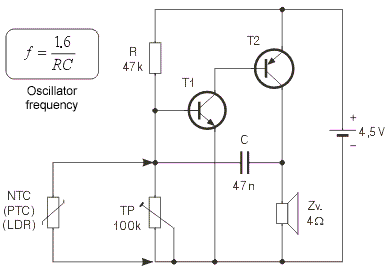Fig. 1.5b: Sound indicator of changes in temperature or the amount of light

Practical use for nonlinear resistors is illustrated on a very simple alarm device, with electrical scheme shown on figure 1.5b. Without trimmer TP and nonlinear NTC resistor it is an audio oscillator. Frequency of the sound it generates can be calculated according to the following formula: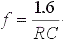In our case, R=47kW and C=47nF, and the frequency equals: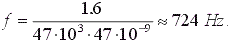When, according to the figure, trimmer pot and NTC resistor are added, oscillator frequency increases but it keeps "playing". If trimmer pot slider is set to the uppermost position, oscillator stops working. At the desired temperature, slider should be lowered very carefully until the oscillator starts working again. For example, if these settings were made at 2°C, oscillator remains still at higher temperatures than that, as NTC resistor's resistance is lower than nominal. If temperature falls the resistance increases and at 2°C oscillator is activated.

If NTC resistor is installed on the car, close to the road surface, oscillator can warn driver if the road is covered with ice. Naturally, resistor and two copper wires connecting it to the circuit should be protected from dirt and water.

If, instead of NTC resistor, PTC resistor is used, oscillator will be activated when temperature rises above certain designated value. For example, PTC resistor could be used for indicating the state of refrigerator: set the oscillator to work at temperatures above 6°C via trimmer TP, and the loud sound will signal if anything's wrong with the fridge.

Instead of NTC, we could use LDR resistor - oscillator would be blocked as long as there is certain amount of light present. In this way, we could make a simple alarm system for rooms where light must be always on.

LDR can be coupled with resistor R. In that case, oscillator works when the light is present, otherwise it is blocked. This could be an interesting alarm clock for huntsmen and fishermen who would like to get up in the crack of dawn, but only if the weather is clear. In the desired moment in the early morning, pot slider should be set to the uppermost position. Then, it should be carefully lowered, until the oscillator is started - this the desired position of the slider. During the night, oscillator will be blocked, since there is no light and LDR resistance is very high. As amount of light increases in the morning, LDR resistance drops and the oscillator is activated when LDR is illuminated with the accurate amount of light matching the previous settings.

Trimmer pot from the figure 1.5b is used for fine adjustments. Aside from that, it can be used for modifying the circuit, if needed. Thus, TP from figure 1.5b can be used for setting the oscillator to activate under different conditions (higher or lower temperature or amount of light).

5. Potentiometers

Potentiometers (also called pots) are variable resistors, used as voltage or current regulators in electronic circuits. By means of construction, they can be divided into 2 groups: coated and coiled.

With coated potentiometers, (figure 1.6a), insulator body is coated with a resistive material. There is an elastic, conductive slider moving across the resistive layer, increasing the resistance between slider and one end of pot, while decreasing the resistance between slider and the other end of pot.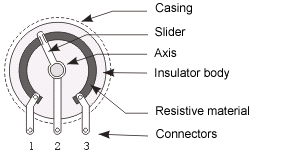Fig. 1.6a: Coated potentiometer

Coiled potentiometers are made of conductor wire coiled around insulator body.  There is an elastic, conductive slider moving across the wire, increasing the resistance between slider and one end of pot, while decreasing the resistance between slider and the other end of pot.

Coated pots are much more common variant. With these, resistance can be linear, logarithmic, inverse-logarithmic or other function depending upon the angle or position of the slider. Most common are linear and logarithmic potentiometers, and the most common applications are radio-receivers, audio amplifiers, and similar devices where pots are used for adjusting the volume, tone, balance, etc.

Coiled potentiometers are used in devices which require increased accuracy and constancy of attributes. They feature higher dissipation than coated pots, and are therefore a necessity in high current circuits.

Potentiometer resistance is commonly of E6 series, most frequently used multipliers including 1, 2.2 and 4.7. Standard tolerance values include 30%, 20%, 10% (and 5% for coiled pots).

Potentiometers come in many different shapes and sizes, with wattage ranging from 1/4W (coated pots for volume control in amps, etc) to tens of Watts (for regulating high currents). Several different pots are shown in the photo 1.6b, along with the symbol for a potentiometer.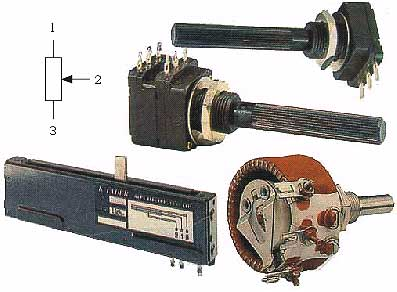Fig. 1.6b: Potentiometers

Uppermost models represent the so called stereo potentiometer. These are actually two pots in one casing, with sliders mounted on shared axis, so they move simultaneously. These are used in stereophonic amps for simultaneous regulation of both LF channels, etc.

Lower left is the so called ruler potentiometer, with a slider moving across straight line, not in circle as with other pots.

Lower right is coiled pot with wattage of 20W, commonly used as rheostat (for regulating current while charging accumulator and similar).

For circuits that demand very accurate voltage and current value, trimmer potentiometers (or just trimmers) are used. These are small potentiometers with slider that is adjusted via screw (unlike other pots where adjustments are made via push-button mounted upon the axis which slider is connected to).

Trimmer potentiometers also come in many different shapes and sizes, with wattage ranging from 0.1W to 0.5W. Image 1.7 shows several different trimmers, along with the symbol for this element.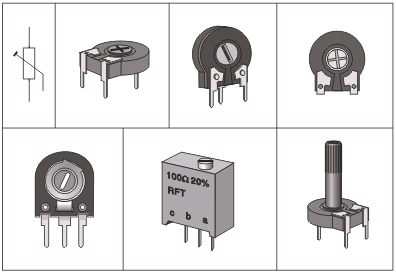Fig. 1.7: Trimmer potentiometers

Resistance adjustments are made via screw. Exception is the trimmer from the lower right corner, which can be also adjusted via plastic axis. Particularly fine adjusting can be achieved with the trimmer in plastic rectangular casing (lower middle). Its slider is moved via special transmission system, so that several full turns of the wheel are required to move slider from one end to the other.

6. Practical examples with potentiometers

As previously stated, potentiometers are most commonly used in amps, radio and TV receivers, cassette players and similar devices. They are used for adjusting volume, tone, balance, etc.

As an example, we will analyze the common circuit for tone regulation in audio amps. It contains two pots and is shown in the figure 1.8a.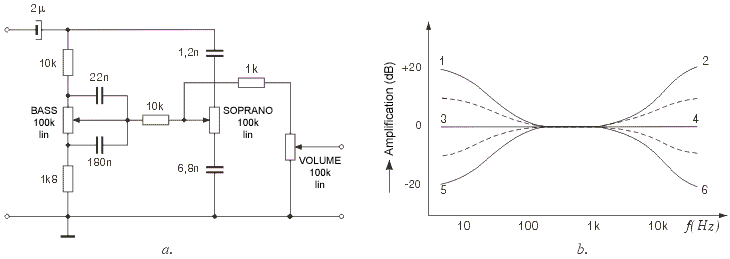Fig. 1.8 Tone regulation circuit: a. Electrical scheme, b. Function of amplification

Potentiometer marked as BASS regulates low frequency amplification. When its slider is in the lowest position, amplification of very low frequency signals (tens of Hz) is about ten times greater than the amplification of mid frequency signals (~kHz). If slider is in the uppermost position, amplification of very low frequency signals is about ten times lower than the amplification of mid frequency signals. Low frequency boost is useful when listening to music with a beat (disco, jazz, R&B...), while LF amplification should be reduced when listening to speech or classical music.

In similar fashion, potentiometer marked as SOPRANO regulates high frequency amplification. High frequency boost is useful when music consists of high-pitched tones such as chimes, while for example HF amplification should be reduced when listening to an old record to reduce the noise.

Diagram 1.8b shows the function of amplification depending upon the signal frequency. If both sliders are in their uppermost position function is described with a curve 1-2, if both are in mid position function is described with a line 3-4, and if both sliders are in their lowest position function is described with a curve 5-6. Setting the pair of sliders to any other possible position results in a curve between curves 1-2 and 5-6.

Potentiometers BASS and SOPRANO are coated by construction and linear by resistance function.

Third pot from the image serves as volume regulator. It is also coated by construction, but is logarithmic by resistance  function (hence the mark log underneath it)

Example with trimmer is given in the text accompanying the image 1.5b.Web Counter by TrafficFile.com